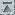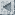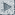## 2.1. Flux Density Scale used at the VLA

```
Careful observations are made with the VLA in the 'D' configuration
every few years to determine the flux densities of 3C286= 1328+307
(B1950), or 1331+305 (J2000); 3C48= 0134+329 (B1950), or 0137+331
(J2000); and 3C147= 0538+498 (B1950) or 0542+498(J2000) relative to
3C295 and the planets.  The flux density scale used at the VLA from
327 MHz to 15 GHz is based on the flux density of 3C295 determined by
Baars et al. (1977 Astron.  Astrophys., 61, 99).  At frequencies above
15 GHz (i.e. the 22 and 43 GHz bands) the flux density scale is based
on observations and emission models for the planet Mars.
In times past a model for the well-studied HII region NGC7027 was used
to set the flux scale at the highest frequencies.  This model is
descrepant with the Mars model by 15% at 43 GHz and a few percent at
22 GHz.  Until this disagreement is understood the flux scale of the
VLA at 22 and 43 GHz should be considered tentative.

According to Baars et al (1977) the flux density of the above sources
at a given frequency is given by the relationship

Log S = A + B * Log v + C * (Log v)**2

where S is the flux density in Jy and v is the frequency in MHz.  The
Baars et al parameters for the sources of interest are

Source         A         B         C

3C48         2.345    +0.071    -0.138
3C147        1.766    +0.447    -0.184
3C286        1.480    +0.292    -0.124
3C295        1.485    +0.759    -0.255  Assumed correct and constant

We assume that the flux density of 3C295 is constant in time and that
its true value is that described by Baars et al. (1977).  This
seems reasonable since its emission is dominated by large (kpc-scale)
hot spots and lobes.  At the highest frequencies this assumption is
not perfectly valid since 3C295 has an inverted spectrum core which is
likely to be variable and is known to contain 0.5% of the flux
density at 15 GHz (Perley & Taylor 1991, AJ 101, 1623).  The
frequency range over which the Baars expressions are valid is 405 MHz
to 15 GHz.  Extrapolation of these expressions to 22 and 45 GHz will
result in a significant error which is why we adopt Mars as the
flux density standard at these frequencies.

```

Go to:ContentsPreviousNext